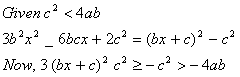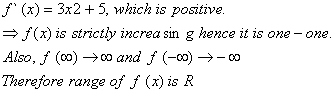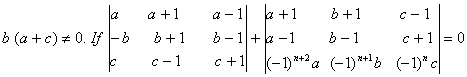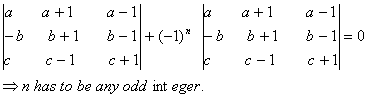Wednesday 17th August 2022
 All India Engineering / Architecture Entrance Examination (AIEEE) CBSE Guess > AIEEE > AIEEE Papers > 2009 > Maths AIEEE 2009 Maths Q. 1. If the roots of the equation bx2 + cx + a = 0 If the roots of the equation be imaginary, then for all real values of x, the expression is less than –4ab greater than 4ab less than 4ab greater than –4ab Sol.:Answer: (4)  Q. 2. For real x, let , f (x) x3 + 5x + 1 then f is neither one-one nor onto R f is one-one but not onto R f is onto R but not one-one f is one-one and onto R Sol:Answer: (4)  Q. 3. Let a, b, c be such that, then the value of n is any integer zero any even integer any odd integer Sol:  The given equation can be written asAnswer: (4)